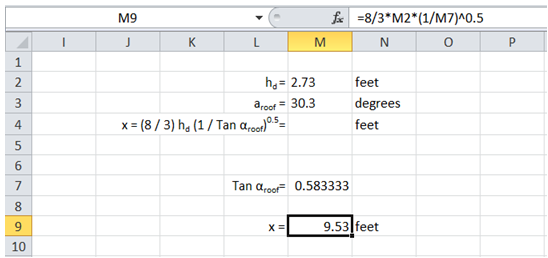## Complex engineering formula in Excel

For example, I need to calculate x using the formula shown below given hd and aroof.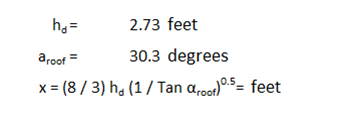To do it in Excel, here is the answer:

a) Break down the formula and work from inside. First determine Tan aroof. To know the function name to be used to calculate this parameter, click on "Math & Trig" under "Formulas" since Tan is a Trigonometry function.

Scroll through the list and select appropriate function as shown below.

Note: The ribbon lists all the categories of functions supported by Excel. Navigating through those lists, select the function of interest as appropriate under general circumstances.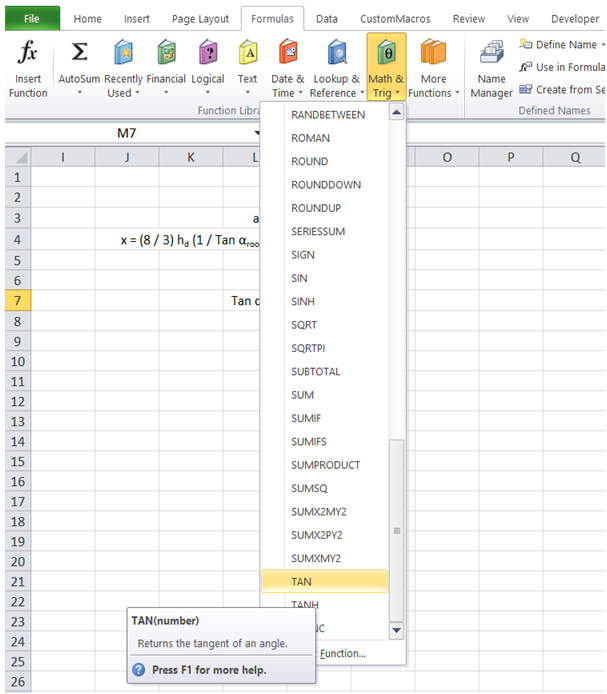b) The "Function Arguments" dialog pops up. In the comments for the function it is clearly mentioned that the angle input for this function should be in radians.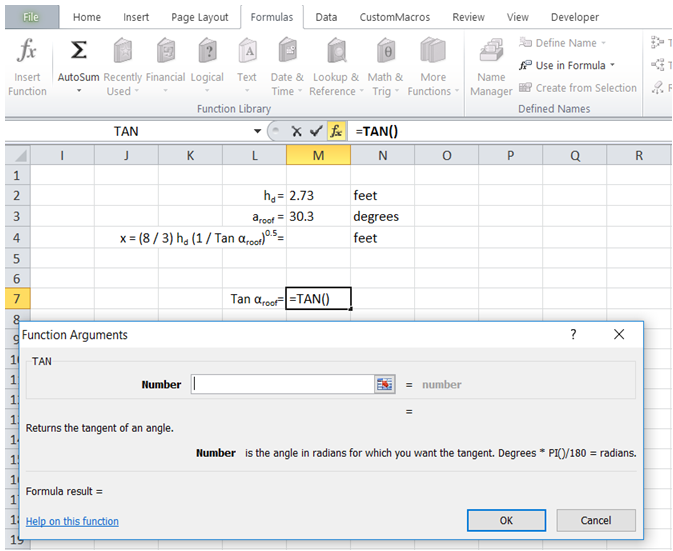c) To convert Degrees to radians, multiply the value by "PI()/180". Alternatively use formula "Radians(M3)" instead of M3*PI()/180.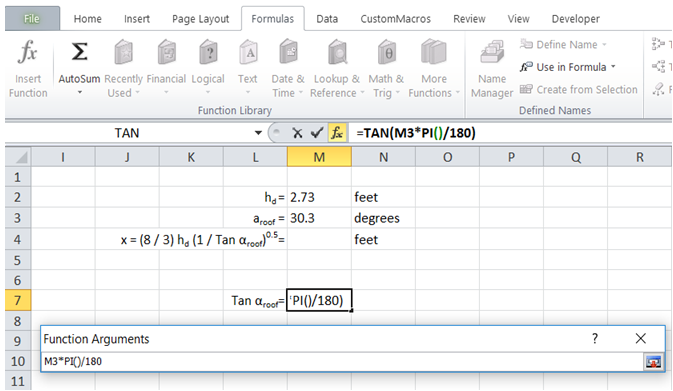d) Tan value is now returned by formula.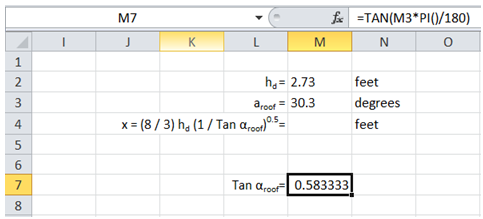e) To get x value, enter the complete formula in a cell as shown below utilizing the Tan aroof value calculated previously.